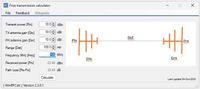# Friss, free space path loss calculator.

Calculates the Free Space Transmission Loss (FSTL) between two antennas based on:

• Transmit power.
• Transmit and receive antenna gains (Gtx & Grx).
• The distance between the two antennas.
• The operating frequency.

Given the parameters above, this tool provides the power at the receive and as Prx.>100 RF Calculators
WinRFCalc, the best RF calculator toolbox for Windows